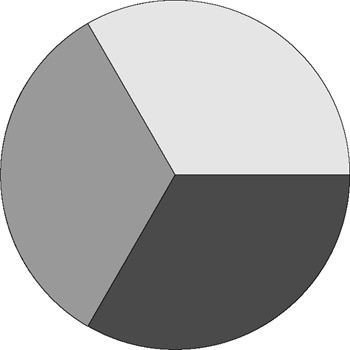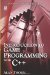# 1.3 Number Parts

## 1.3 Number Parts

It has been demonstrated using basic arithmetic that numbers can be added, subtracted, multiplied, and divided to produce other numbers, some of which are larger or smaller than others. On closer inspection it will be seen that every countable number is measured by its relationship to 1. So 2 is twice as large as 1, 3 is three times as large as 1, and so on. For this reason, a smaller number is said to be part of a larger number, and the larger number is said to be a multiple of the smaller. A part is called an aliquot part if the smaller number is contained inside the larger an exact number of times. In modern times, this smaller number has come to be called a factor of the larger number (multiple). Consider the following multiples of 2:

• 2, 4, 6, 8, 10, 12, 14, 16, 18

Here, 2 is the first multiple, 4 is the second, 6 is the next, and so it continues. The number 2 has a relationship with each of its multiples. It can be said to be a part of each of these numbers, except itself. 14 is the seventh multiple of 2, and therefore 2 is the seventh part of 14. Following this logic, 2 is the fifth part of 10 and the fourth part of 8. Rather than saying 2 is the second part of 4, we tend to state that 2 is half of 4. However, in most of these cases it should be noted that in order to describe how 2-or any number-relates to its multiples, we use the ordinal numbers to do so; for example, 2 is the eighth part of 16.Figure 1.1Introduction to Game Programming with C++ (Wordware Game Developers Library)
ISBN: 1598220322
EAN: 2147483647
Year: 2007
Pages: 225
Authors: Alan Thorn

Similar book on Amazon

flylib.com © 2008-2017.
If you may any questions please contact us: flylib@qtcs.net